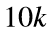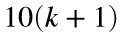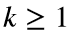# Problem 52183. List the nth prime quartet prefix

Prime numbers larger than 5 can end only in 1, 3, 7, or 9, but of course not all numbers ending in these four digits are prime. Let’s call a group of four prime numbers betweenand(with k an integer and) a prime quartet. The first one is 11, 13, 17, and 19, and the second is 101, 103, 107, and 109. Therefore, the prefix (or the number excluding the last digit) of the first prime quartet is 1, and the prefix of the second prime quartet is 10.
Write a function that returns the nth prime quartet prefix.
Optional: Prove that the sequence of prime quartet prefixes is infinite. Just drop your proof in the comments below.

### Solution Stats

15.38% Correct | 84.62% Incorrect
Last Solution submitted on May 02, 2023

### Community Treasure Hunt

Find the treasures in MATLAB Central and discover how the community can help you!

Start Hunting!